Courses

# Test: Thermodynamic (Level - II)- 1

## 25 Questions MCQ Test Mechanical Engineering SSC JE (Technical) | Test: Thermodynamic (Level - II)- 1

Description
This mock test of Test: Thermodynamic (Level - II)- 1 for Mechanical Engineering helps you for every Mechanical Engineering entrance exam. This contains 25 Multiple Choice Questions for Mechanical Engineering Test: Thermodynamic (Level - II)- 1 (mcq) to study with solutions a complete question bank. The solved questions answers in this Test: Thermodynamic (Level - II)- 1 quiz give you a good mix of easy questions and tough questions. Mechanical Engineering students definitely take this Test: Thermodynamic (Level - II)- 1 exercise for a better result in the exam. You can find other Test: Thermodynamic (Level - II)- 1 extra questions, long questions & short questions for Mechanical Engineering on EduRev as well by searching above.
QUESTION: 1

Solution:
QUESTION: 2

Solution:
QUESTION: 3

### Match the following List- I (Thermometer) with List -II (Thermometric Property):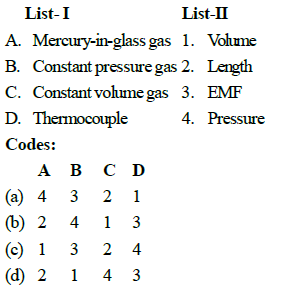Solution:
QUESTION: 4

Convert the following reading of pressure to kPa, assuming that the barometer reads 760 mm of Hg and match the List-I with List-II: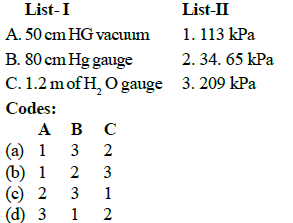Solution:
QUESTION: 5

Which of the following aspect is not true regarding microscopic properties of thermodynamic system

Solution:
QUESTION: 6

Choose the correct statement among the following:

Solution:
QUESTION: 7

Consider the following:

1. Temperature

2. Viscosity3. Specific entropy

4. Thermal conductivity

Which of the above properties of a system is/are intensive?

Solution:
QUESTION: 8

The value of an extensive property is extensively dependent on

Solution:
QUESTION: 9

which of the following are intensive properties
1. Kinetic energy                2. Specific enthalpy
3. Pressure                         4. Entropy

Solution:
QUESTION: 10

which of the following statements regarding the concept of continuum are correct?

1. Large number of molecules enable meaningful statistical averaging and assignment of property values

2. Mean free path of the molecules is order of magnitude higher than system dimensions

3. Behaviour of individual molecules is disregarded

4. Mean free path of the molecules approaches the order of magnitude of the system dimensions

Solution:

Explanation : Concept of Continuum is a kind of idealization of the continuous description of matter where the properties of matter are considered as continuous function of space variables. Although any matter is composed of several molecules, the concept of continuum assumes a continuous distribution of mass within the matter or system with no empty space, instead of actual conglomeration of separate molecules (a thing consisting of number of different distinct parts or item and that are grouped together it is meaning of conglomeration) for follow continuum concept characteristic length of molecular system should be more than mean free path (distance between two collisions) characteristic length is diameter of molecule < D (follow continuum concept).

QUESTION: 11

Match the following List - I (Type of thermometer) with List -II (Thermometric property) the following :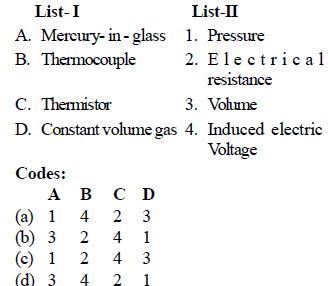Solution:
QUESTION: 12

Experimental data obtained from a constantvolume gas thermometer is shown is the figure below. The value of l in ºC is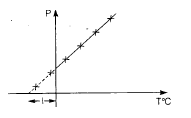Solution:
QUESTION: 13

The critical point and triple point data for water are
Tc = 374 ºC, Pc = 22.1 MPa
TT = 0.01 ºC PT = 0.6 KPa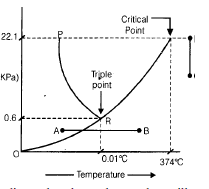Indicate the phase change that will occur in following cases:
(i) Ice at 0.5 Kpa is heated isobarically
(ii) Water vapour at 400ºC is compressed isothermally

Solution:
QUESTION: 14

A closed system receives 60 kJ heat but its internal energy decreases by 30 kJ. Then the work done by the system is

Solution:
QUESTION: 15

In a general compression process, 2 kJ of mechanical work is supplied to 4 kg of fluid and  800 J of heat is rejected to the cooling jacket. The change in specific internal energy would be

Solution:
QUESTION: 16

A paddle wheel used for stirring a liquid contained in a tank supplied 5000 kJ of work and during the stirring operation the tank lost 1500 kJ of heat to the surroundings. If the tank and liquid are considered as a system the change in its internal energy will be

Solution:
QUESTION: 17

Which one of the following statement bolds good for the equation dQ=dE +dW

Solution:
QUESTION: 18

300 kJ/s of heat is supplied at a constant fixed temperature of 290ºC to a heat engine. The heat rejection takes place at 8.5ºC. Then match the following.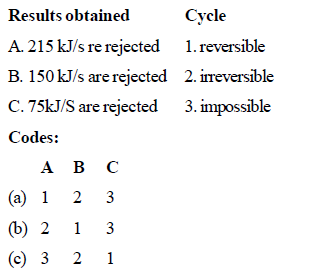Solution:
QUESTION: 19

Match List-I with List-II and select the correct answer using the codes given below.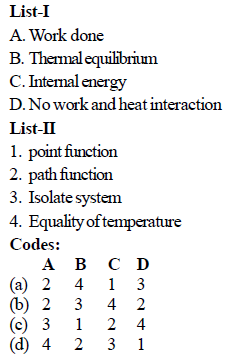Solution:
QUESTION: 20

Match the following :
A. Heat   I. State function
B. Internal energy   II. Path function
C.Work
D. Entropy

Solution:
QUESTION: 21

In a cyclic process heat transfer are + 15.7 kJ, - 26.2 kJ, – 4.86 kJ and + 31.5 kJ. What is the net work for this cyclic process?

Solution:
QUESTION: 22

The value of heat and work transfer for the flow processes of a thermodynamic cycle are given below: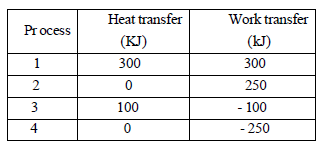The thermal efficiency and work ratio for the cycle will be respectively

Solution:
QUESTION: 23

According to first law of thermodynamics

Solution:
QUESTION: 24

During a thermodynamic process, 84 kJ of heat flows into the system and the work done by the system is 32 kJ. The increase in internal energy of the system is

Solution:
QUESTION: 25

Given that along the path 1-2-3 a system absorbs 100 kJ as heat and does 60 kJ work while along the pth 1-4-3 it does 20 kJ work (see figure given). The heat absorbed during the cycle 1-4-3 is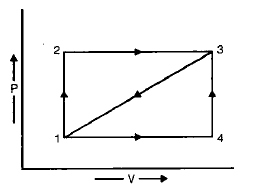Solution: